# The Runcinated 5-cell

The runcinated 5-cell is a uniform polychoron bounded by 30 cells (10 tetrahedra and 20 triangular prisms), 70 faces (40 triangles and 30 squares), 60 edges, and 20 vertices. It is constructed by radially expanding the 5 cells of the pentachoron outwards, and filling in the resulting gaps with triangular prisms and 5 more tetrahedra.

## Structure

We will explore the structure of the runcinated 5-cell by means of its parallel projection into 3D.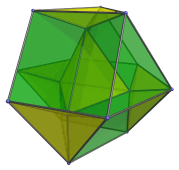The projection envelope is a cuboctahedron. For clarity, we've omitted the cells that lie on the far side.

### Northern Hemisphere

The tetrahedron at the center of the projection is the closest cell to the 4D viewpoint. We may call it the “North Pole” cell. We show only this cell in the following image: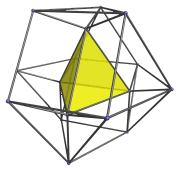Each of its 4 triangular faces is joined to a triangular prism: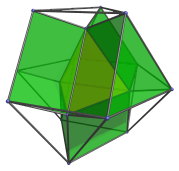Six more triangular prisms sit between each pair of these prisms: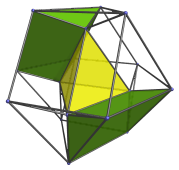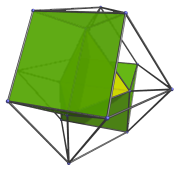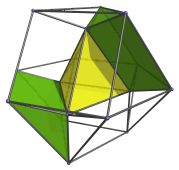Finally, there are 4 tetrahedral cells that touch the central cell's vertices: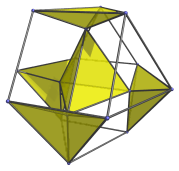These are all the cells on the “northern hemisphere” of the runcinated 5-cell.

### Southern Hemisphere

Now we come to the southern hemisphere of the runcinated 5-cell. There are also 4 tetrahedral cells that touch the “South Pole” cell. However, they are in dual orientation from the cells we last saw in the northern hemisphere: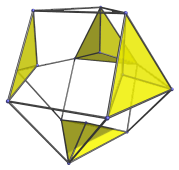For clarity, we've omitted the edges and vertices that belong to the northern hemisphere.

The South Pole cell itself is also in dual orientation to the North Pole cell: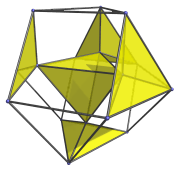Six triangular prisms touch the edges of the South Pole cell: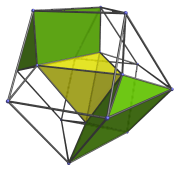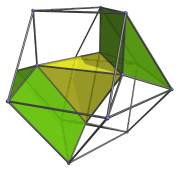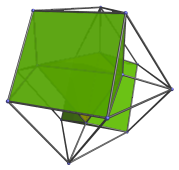And finally, we show the 4 triangular prisms that share a face with the South Pole cell: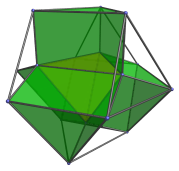### Summary

In total, the northern hemisphere has 1 North Pole cell + 4 tetrahedra, making 5 tetrahedra, with 4+6=10 triangular prisms. The southern hemisphere likewise has 1 South Pole cell + 4 tetrahedra, with 6+4=10 triangular prisms. All in all, we have 10 tetrahedra and 20 triangular prisms.

## Properties

The runcinated 5-cell has some interesting properties. Its two hemispheres can each be considered the 4D equivalent of a cupola. In 3D, for example, the triangular cupola may be constructed by starting with a triangle, and sweeping out a 3D volume as we pull its edges apart gradually until it becomes a hexagon. This produces a solid bounded by 1 triangle at the top, 3 squares and 3 triangles around its slope, and a hexagon at the bottom. Interestingly enough, two triangular cupolas joined base-to-base, each in dual orientation to the other, forms a cuboctahedron, which is the shape of the projection envelope of the runcinated 5-cell.

This is no mere coincidence; if we now take a tetrahedron (the 3D equivalent of a triangle) and sweep out a 4D volume as we pull its faces apart, it eventually becomes a cuboctahedron. As we pull the tetrahedron apart, its vertices expand into triangles, which trace out tetrahedra as we sweep out the 4D volume. Its faces trace out triangular prisms, and its edges likewise. Thus, the resulting 4D solid is bounded by 1 tetrahedron + 4 other tetrahedra, 4 + 6 triangular prisms (corresponding with the original tetrahedron's faces and edges, respectively), and a cuboctahedron at the bottom. We may, by analogy, call this the tetrahedral cupola, and we observe that it is exactly one of the hemispheres of the runcinated 5-cell.

This pattern in fact generalizes to any number of dimensions. If we begin with a pentachoron, and trace out a 5D volume as we pull its cells apart, we get a 5D pentachoron cupola, two of which can be joined base-to-base in dual orientation to form the 5D equivalent of the runcinated 5-cell. Starting with a 5D simplex, we can construct a 6D simplicial cupola, two of which can be joined together in dual orientation (this is always possible because the n-dimensional simplex is always self-dual) to form the 6D expanded simplex, and so forth.

## Coordinates

The Cartesian coordinates of the runcinated 5-cell, centered on the origin and having edge length 2, are:

• (0, 0, 0, ±2)
• (0, 0, ±3/√3, ±1)
• ±(0, 4/√6, −2/√3, 0)
• ±(0, 4/√6, 1/√3, ±1)
• ±(5/√10, −3/√6, 0, 0)
• ±(5/√10, 1/√6, −2/√3, 0)
• ±(5/√10, 1/√6, 1/√3, ±1)

Simpler coordinates can be obtained in 5D as all permutations of coordinates of:

• (0, √2, √2, √2, 2√2)

The 4D coordinates are derived by projecting these 5D coordinates back into 4D using a symmetric projection.

Last updated 17 Jun 2019.